# Colleges with the highest SAT scores in Mississippi

Top 8 colleges in Mississippi with the highest SAT scores
Looking for the colleges with the highest SAT scores in Mississippi? Well you're in luck! We've compiled a national college database and have created a list of the top 8 universities with the highest SAT scores in Mississippi. These are the schools whose applicants had the highest average SAT scores in Mississippi. And, since these tests are meant to determine academic prowess, they are arguably the schools with the most academically proficient students. You could even say these are the best colleges in Mississippi. We also include each college's ACT scores and acceptance rate so that you can see where you would have the easiest or hardest time getting in. Read on to find out more.

## Mississippi State University SAT scores

The average SAT score for Mississippi State University is 1180.The average SAT score of 1180 breaks down into:

• SAT math: 580

The average ACT score for Mississippi State University is 25 and their acceptance rate is 73.4%.

## The University of Mississippi SAT scores

The average SAT score for The University of Mississippi is 1160.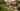The average SAT score of 1160 breaks down into:

• SAT math: 575

The average ACT score for The University of Mississippi is 25 and their acceptance rate is 88.1%.

## Belhaven University SAT scores

The average SAT score for Belhaven University is 1123.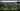The average SAT score of 1123 breaks down into:

• SAT math: 550

The average ACT score for Belhaven University is 22 and their acceptance rate is 59.1%.

## William Carey University SAT scores

The average SAT score for William Carey University is 1090.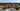The average SAT score of 1090 breaks down into:

• SAT math: 590

The average ACT score for William Carey University is 25 and their acceptance rate is 93%.

## University of Southern Mississippi SAT scores

The average SAT score for University of Southern Mississippi is 1070.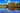The average SAT score of 1070 breaks down into:

• SAT math: 530

The average ACT score for University of Southern Mississippi is 23 and their acceptance rate is 98.5%.

## Delta State University SAT scores

The average SAT score for Delta State University is 1067.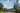The average SAT score of 1067 breaks down into:

• SAT math: 540

The average ACT score for Delta State University is 21 and their acceptance rate is 85%.

## Alcorn State University SAT scores

The average SAT score for Alcorn State University is 970.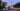The average SAT score of 970 breaks down into:

• SAT math: 490

The average ACT score for Alcorn State University is 19 and their acceptance rate is 78.6%.

## Jackson State University SAT scores

The average SAT score for Jackson State University is 930.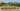The average SAT score of 930 breaks down into:

• SAT math: 465